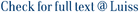We study the problem of producing hierarchical drawings of layered graphs when some pairs of edges are not allowed to cross. We show that deciding on the existence of a drawing satisfying at least k constraints from a given set of non-crossing constraints is NP-complete even if the graph is 2-layered and even when the permutation of the vertices on one side of the bipartition is fixed. We also propose simple constant-ratio approximation algorithms for the optimization version of the problem and we discuss how to extend the well-known hierarchical approach for creating layered drawings of directed graphs with the capability of minimizing the number of edge crossings while maximizing the number of satisfied non-crossing constraints.

Layered drawings of graphs with crossing constraints / Finocchi, Irene. - Proc. of the 7th Annual Int. Computing and Combinatorics Conference (COCOON'01), (2001), pp. 357-367. (7th Annual International Conference on Computing and Combinatorics, GUILIN, PEOPLES R CHINA, AUG 20-23, 2001). [10.1007/3-540-44679-6_39].

### Layered drawings of graphs with crossing constraints

#### Abstract

We study the problem of producing hierarchical drawings of layered graphs when some pairs of edges are not allowed to cross. We show that deciding on the existence of a drawing satisfying at least k constraints from a given set of non-crossing constraints is NP-complete even if the graph is 2-layered and even when the permutation of the vertices on one side of the bipartition is fixed. We also propose simple constant-ratio approximation algorithms for the optimization version of the problem and we discuss how to extend the well-known hierarchical approach for creating layered drawings of directed graphs with the capability of minimizing the number of edge crossings while maximizing the number of satisfied non-crossing constraints.
##### Scheda breve Scheda completa Scheda completa (DC)2001
9783540424949
File in questo prodotto:
Non ci sono file associati a questo prodotto.
##### Pubblicazioni consigliate

I documenti in IRIS sono protetti da copyright e tutti i diritti sono riservati, salvo diversa indicazione.

Utilizza questo identificativo per citare o creare un link a questo documento: `https://hdl.handle.net/11385/192537`
##### Citazioni
•6
•4